Fourier Series: Using The Derived Formulas for Determination of Fourier Foefficients

First we will "do it the hard way" and write short programs that evaluate Fourier coefficients; then we will demonstrate how to make use of built-in functions in Mathematica's FourierTransform package…

Define functions based on the formulas derived for the fourier amplitudes

The constant term: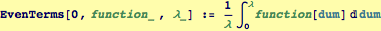A function that defines each even amplitude individually (this is not very efficient, it would be better to evaluate the integral once and use that result)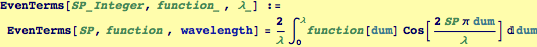Define the zeroth odd term as zero for symmetry with the even terms: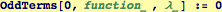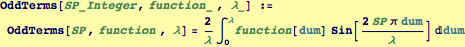A function to create a vector of amplitudes for the odd terms and one for the even terms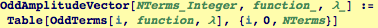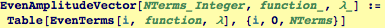Created by Wolfram Mathematica 6.0  (24 October 2007)